# Practical Exercise 7: THE MOON AGAIN

ahe measurement showed that the angular size (average) of the Moon in perigee is θ☽per = 33,5' and in apogee is θ☽per = 33,5' and in apogee is θ☽apo  =  29,9'.ahe radius of the Moon is θ☽per = 33,5' and in apogee it is θ☽apo = 29,9'. and in apogee it is R = 1 737 km, determine the numerical eccentricity of the elliptic orbit and its main half-axis.

The key is to start from the definition of angular size: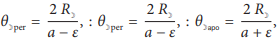a teda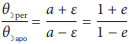By modifying the last equation we get: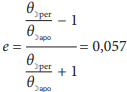.

ahe actual value of the numerical eccentricity is 0,055. The trajectory is therefore almost circular.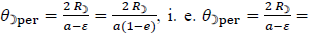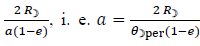where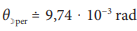. We determine the main half-axis based on this relation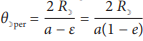, i.e.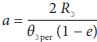, where. After substitution we get a ≐ 378 000 km. We can check the result by using the second relation (for apogee):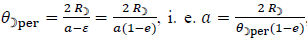, kde. After substitution we get a ≐ 378 000 km. VWe can check the result by using the second relation (for apogee):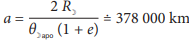, where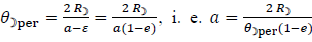, where. After substitution we get a ≐ 378 000 km.

We can check the result by using the second relation (for apogee):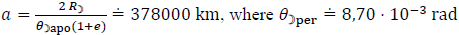.